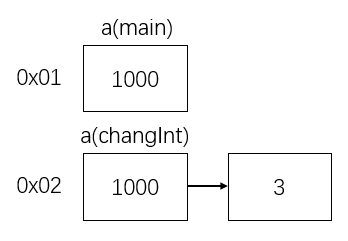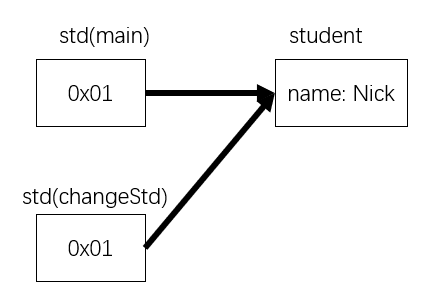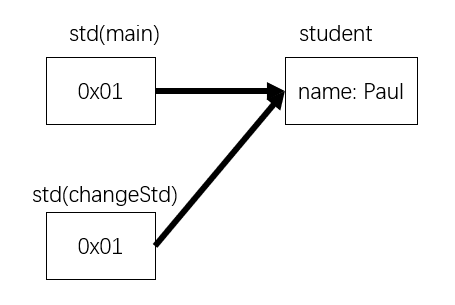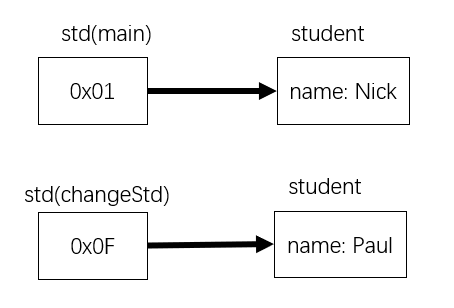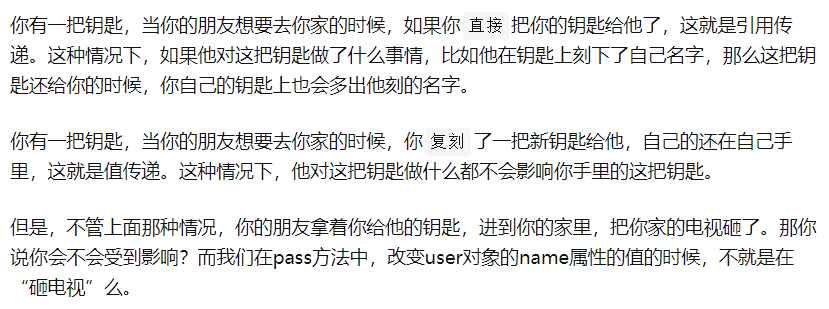# Java 值传递 or 引用传递？

## 值传递 or 引用传递？

• 值传递（pass by value）：是指在调用函数时将实际参数复制一份传递到函数中，这样在函数中如果对参数进行修改，将不会影响到实际参数
• 引用传递（pass by reference）：是指在调用函数时将实际参数的地址直接传递到函数中，那么在函数中对参数所进行的修改，将影响到实际参数

Java的数据类型分为两类

• 基本类型（int float等）
• 引用类型（string, 数组等, 以及一切类对象）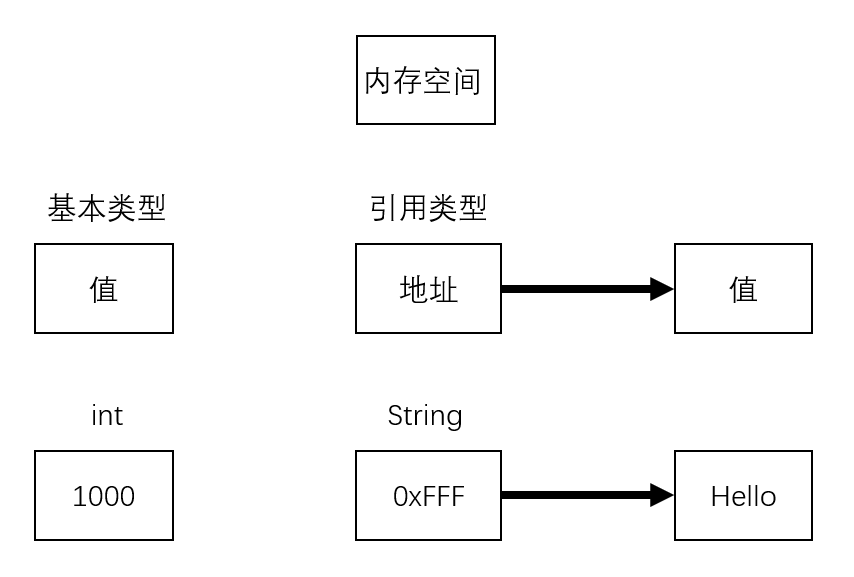``````public class test {

public static void main(String[] args) {
int a = 1000;
changeInt(a);
System.out.println("(main)a = " + a);
}

private static void changeInt(int a) {
a = 3;
System.out.println("(changeInt)a = " + a);
}
}

// 运行代码会得到如下结果
// (changeInt)a = 3
// (main)a = 1000
``````

``````public class test {
public static void main(String[] args) {
Student std = new Student();
std.name = "Nick";
changeStd(std);
System.out.println("(main)name = " + std.name);
}

private static void changeStd(Student std) {
std.name = "Paul";
System.out.println("(change)name = " + std.name);
}
}

class Student {
String name;
}

// 运行结果
// (change)name = Paul
// (main)name = Paul
``````

``````public class test {
public static void main(String[] args) {
Student std = new Student();
std.name = "Nick";
changeStd(std);
System.out.println("(main)name = " + std.name);
}

private static void changeStd(Student std) {
std = new Student();
std.name = "Paul";
System.out.println("(change)name = " + std.name);
}
}

class Student {
String name;
}

// 运行结果
// (change)name = Paul
// (main)name = Nick
``````